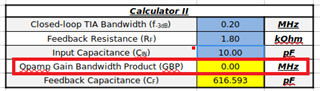If you have a related question, please click the "Ask a related question" button in the top right corner. The newly created question will be automatically linked to this question.

# Min GBP for TIA, SBOA122

Hi!

Is Eq. 12 for min GBP with a Butterworth response correct in sboa122.pdf?

Example, required:

Transimpedance is 1800 V/A, Frequency at -3 dB is 200k, Cin = 10 pF.

Compute the circuit values:

The feedback resistor is 1800 Ohm, the feedback capacitor is 430 pF.

According eq. 12:

GBP = 2 * pi * 200 kHz^2 * 1800 * 10 pF = 4500 Hz

• Hello Dmil,

This equation is an estimate of the required gain bandwidth product for a TIA to achieve a closed loop bandwidth with a Butterworth response or with Q = 0.707 (phase margin of 65 degrees) or maximally flat pass-band.

A full transfer function of a TIA can be found which would give an exact number/relationship at different qualify factors, but equation 12 is a good way to quickly and efficiently estimate the needed GBP for any given TIA requirements.

We have a TIA calculator that can give the above and also give you the reverse which is maximum closed loop bandwidth from a given amplifiers GBP. It can be found at this link: https://e2e.ti.com/support/amplifiers-group/amplifiers/f/amplifiers-forum/956151/faq-transimpedance-amplifier-calculator

Thank you,

Sima

• Hello Sima,

Could you give an example how to use this equation if a bandwidth of a TIA is specified?

Also, your calculator looks strange. I do not see how to compute TIA component values to get desired input parameters.

And when I entered known values I got a strange result, see the picture.Thank you,

Dmil

• Hello Dmil,

It looks like it is computing too small of a GBP for the calculator. If you increase to 0.3MHz for the closed loop bandwidth, you will see 0.01MHz GBP which is the smallest value this calculator can compute.

The calculator does not compute input TIA parameters. but It is possible to back-calculate for these values with the equation.

Thank you,

Sima

• Hello Sima,

It is clear that GBP of 10 kHz is incorrect value for a TIA with 300 kHz bandwidth.

Best regards,

Dmitriy

• Hi Dmitriy,

What is happening with your case is that your bandwidth is low enough and the input capacitance is small enough that we actually need a Cf larger than Cin to compensate the circuit to 200kHz bandwidth. Unfortunately that confuses the calculations in the spreadsheet which is why you are getting strange results.

For your case you can start with an amplifier that is just greater than your target bandwidth. However, the amplifier's input capacitance will add to your total input capacitance which may change the equation slightly. I would suggest simulating in SPICE to verify the circuit bandwidth. \

Regards,

Jacob

• Hi Jacob,

The situation when Cf is larger than Cin is common.

I think, some changes should be done in sboa122 and the calculator.

Best regards,

Dmil

• Hi Dmil,

Thank you for the feedback, we will work to add this case to the calculator.

Best,

Jacob